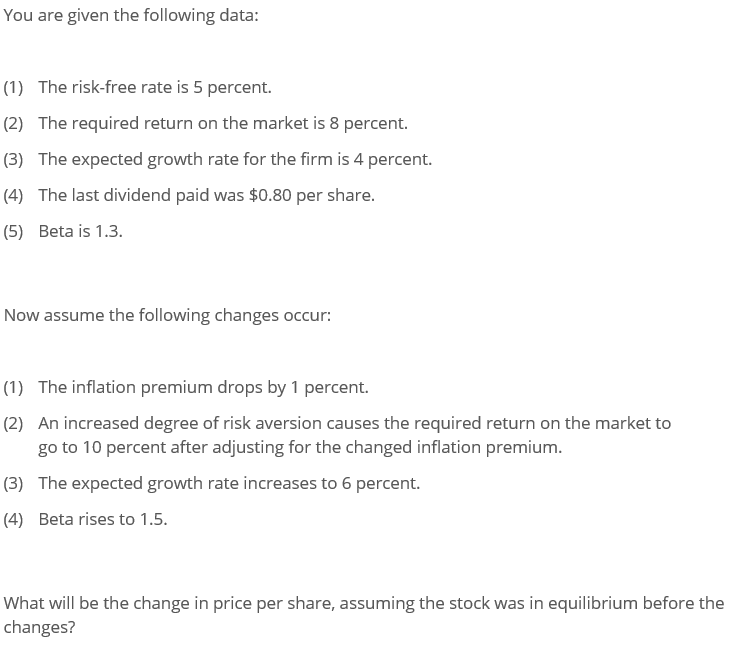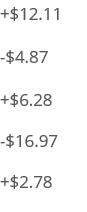Home / Answered Questions / Other / you-are-given-the-following-data-1-the-risk-free-rate-is-5-percent-2-the-required-return-on-the-mark-aw442

# (Solved): You Are Given The Following Data: (1) The Risk-free Rate Is 5 Percent. (2) The Required Return On Th...please show work in written form (not excel) thank you.

You are given the following data: (1) The risk-free rate is 5 percent. (2) The required return on the market is 8 percent. (3) The expected growth rate for the firm is 4 percent. (4) The last dividend paid was \$0.80 per share. (5) Beta is 1.3. Now assume the following changes occur: (1) The inflation premium drops by 1 percent. (2) An increased degree of risk aversion causes the required return on the market to go to 10 percent after adjusting for the changed inflation premium. (3) The expected growth rate increases to 6 percent. (4) Beta rises to 1.5. go What will be the change in price per share, assuming the stock was in equilibrium before the changes? +\$12.11 -\$4.87 +\$6.28 -\$16.97 +2.78

We have an Answer from Expert